Cross Reference Up

Duncan, RL (1967). An application of uniform distributions to the Fibonacci numbers. Fibonacci Quarterly 5, pp. 137-140.

This work is cited by the following items of the Benford Online Bibliography:

Note that this list may be incomplete, and is currently being updated. Please check again at a later date.

 Berger, A and Eshun, G (2014). Benford solutions of linear difference equations. Theory and Applications of Difference Equations and Discrete Dynamical Systems, Springer Proceedings in Mathematics & Statistics Volume 102, pp. 23-60. ISSN/ISBN:978-3-662-44139-8. DOI:10.1007/978-3-662-44140-4_2.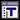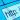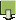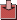Berger, A and Eshun, G (2016). A characterization of Benford's law in discrete-time linear systems. Journal of Dynamics and Differential Equations 28(2), pp. 432-469. ISSN/ISBN:1040-7294. DOI:10.1007/s10884-014-9393-y.Jager, H and Liardet, P (1988). Distribution arithmétiques des dénominateurs de convergents de fractions continues. Nederl. Akad. Wetensch. Indag. Math. 50(2), pp. 181-197. DOI:10.1016/S1385-7258(88)80026-X. FREKanemitsu, S, Nagasaka, K, Rauzy, G and Shiue, JS (1988). On Benfords law: the first digit problem. Lecture Notes in Mathematics 1299, pp. 158-169 (eds. Watanabe, S, and Prokhorov, YV). ISSN/ISBN:978-3-540-18814-8. DOI:10.1007/BFb0078471.Khosravani, A and Rasinariu, C (2015). n-digit Benford converges to Benford. Int. J. Math. Math. Sci. 2015, Art. ID 123816, 4 pp. 60F25 (11K45). DOI:10.1155/2015/123816.Kuipers, L (1969). Remark on a paper by R.L. Duncan concerning the uniform distribution mod 1 of the sequence of the logarithms of the Fibonacci numbers. Fibonacci Quarterly 7, pp. 465-466, 473.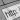Nagasaka, K (1984). On Benford's Law. Annals of the Institute of Statistical Mathematics 36(2), pp. 337-352. ISSN/ISBN:0020-3157. DOI:10.1007/BF02481974.Nagasaka, K, Kanemitsu, S and Shiue, JS (1990). Benfords law: The logarithmic law of first digit. In: Győry, K, Halász, G. (eds.) Number theory. Vol. I. Elementary and analytic, Proc. Conf., Budapest/Hung. 1987, Colloq. Math. Soc. János Bolyai 51, pp. 361-391 .Nigrini, MJ (1992). The Detection of Income Tax Evasion Through an Analysis of Digital Frequencies. PhD thesis, University of Cincinnati, OH, USA.Pollach, G, Brunkhorst, F, Mipando, M, Namboya, F, Mndolo, S and Luiz, T (2016). The "first digit law" - A hypothesis on its possible impact on medicine and development aid. Medical Hypotheses 97, pp. 102-106. DOI:10.1016/j.mehy.2016.10.021.Raimi, RA (1976). The First Digit Problem. American Mathematical Monthly 83(7), pp. 521-538. ISSN/ISBN:0002-9890. DOI:10.2307/2319349.